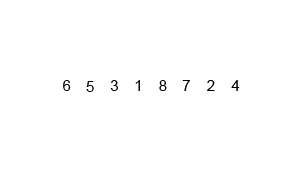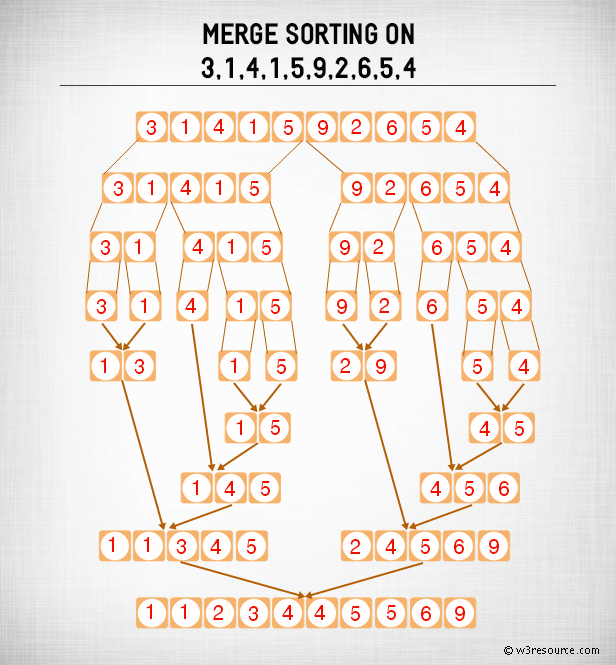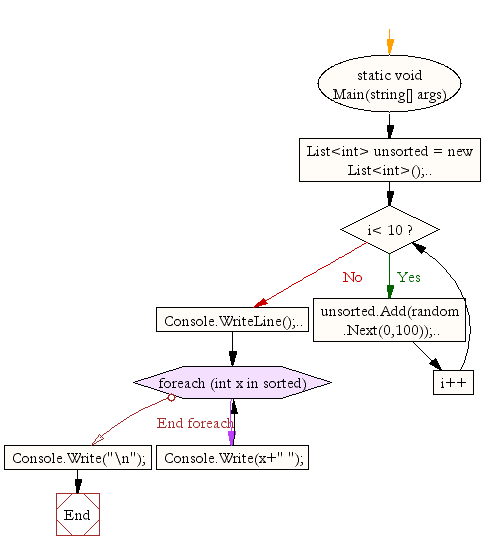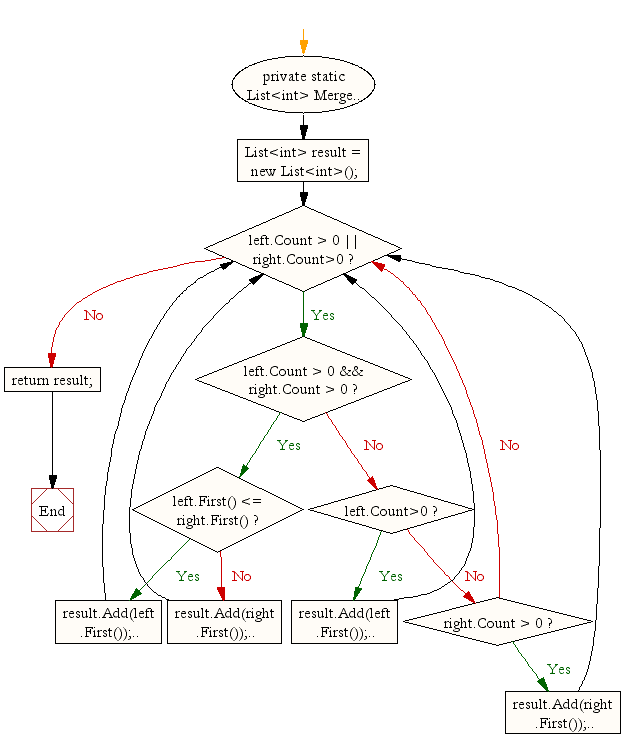﻿ C# - Merge sort

# C#: Merge sort

## C# Sharp Searching and Sorting Algorithm: Exercise-7 with Solution

Write a C# Sharp program to sort a list of elements using Merge sort.

According to Wikipedia "Merge sort (also commonly spelled mergesort) is an O (n log n) comparison-based sorting algorithm. Most implementations produce a stable sort, which means that the implementation preserves the input order of equal elements in the sorted output."

Algorithm :

Conceptually, a merge sort works as follows :

• Divide the unsorted list into n sublists, each containing 1 element (a list of 1 element is considered sorted).
• Repeatedly merge sublists to produce new sorted sublists until there is only 1 sublist remaining. This will be the sorted list.

An example of merge sort :Merge Sort: Pictorial PresentationSample Solution:-

C# Sharp Code:

``````using System;
using System.Collections.Generic;
using System.Linq;
using System.Text;

namespace Merge_sort
{
class Program
{
static void Main(string[] args)
{
List<int> unsorted = new List<int>();
List<int> sorted;

Random random = new Random();

Console.WriteLine("Original array elements:" );
for(int i = 0; i< 10;i++){
Console.Write(unsorted[i]+" ");
}
Console.WriteLine();

sorted = MergeSort(unsorted);

Console.WriteLine("Sorted array elements: ");
foreach (int x in sorted)
{
Console.Write(x+" ");
}
Console.Write("\n");
}

private static List<int> MergeSort(List<int> unsorted)
{
if (unsorted.Count <= 1)
return unsorted;

List<int> left = new List<int>();
List<int> right = new List<int>();

int middle = unsorted.Count / 2;
for (int i = 0; i < middle;i++)  //Dividing the unsorted list
{
}
for (int i = middle; i < unsorted.Count; i++)
{
}

left = MergeSort(left);
right = MergeSort(right);
return Merge(left, right);
}

private static List<int> Merge(List<int> left, List<int> right)
{
List<int> result = new List<int>();

while(left.Count > 0 || right.Count>0)
{
if (left.Count > 0 && right.Count > 0)
{
if (left.First() <= right.First())  //Comparing First two elements to see which is smaller
{
left.Remove(left.First());      //Rest of the list minus the first element
}
else
{
right.Remove(right.First());
}
}
else if(left.Count>0)
{
left.Remove(left.First());
}
else if (right.Count > 0)
{

right.Remove(right.First());
}
}
return result;
}
}
}
```
```

Sample Output:

```Original array elements:
79 69 9 95 65 49 65 40 27 95
Sorted array elements:
9 27 40 49 65 65 69 79 95 95
```

Flowchart:C# Sharp Code Editor: